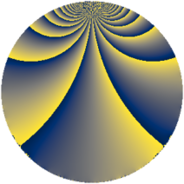# Properties

 Label 475.3.zLevel $475$ Weight $3$ Character orbit 475.z Rep. character $\chi_{475}(69,\cdot)$ Character field $\Q(\zeta_{30})$ Dimension $784$ Sturm bound $150$

# Related objects

## Defining parameters

 Level: $$N$$ $$=$$ $$475 = 5^{2} \cdot 19$$ Weight: $$k$$ $$=$$ $$3$$ Character orbit: $$[\chi]$$ $$=$$ 475.z (of order $$30$$ and degree $$8$$) Character conductor: $$\operatorname{cond}(\chi)$$ $$=$$ $$475$$ Character field: $$\Q(\zeta_{30})$$ Sturm bound: $$150$$

## Dimensions

The following table gives the dimensions of various subspaces of $$M_{3}(475, [\chi])$$.

Total New Old
Modular forms 816 816 0
Cusp forms 784 784 0
Eisenstein series 32 32 0

## Trace form

 $$784q - 15q^{2} - 15q^{3} + 189q^{4} - 3q^{5} - 19q^{6} + 279q^{9} + O(q^{10})$$ $$784q - 15q^{2} - 15q^{3} + 189q^{4} - 3q^{5} - 19q^{6} + 279q^{9} - 39q^{10} - 42q^{11} - 15q^{13} - 63q^{14} + 111q^{15} + 373q^{16} - 40q^{17} + 24q^{19} - 8q^{20} + 72q^{21} - 135q^{22} + 65q^{23} + 24q^{24} + 51q^{25} - 148q^{26} + 75q^{28} - 99q^{29} + 256q^{30} - 285q^{33} - 9q^{34} + 181q^{35} + 467q^{36} - 655q^{38} - 288q^{39} + 636q^{40} - 9q^{41} + 175q^{42} - 277q^{44} + 768q^{45} - 115q^{47} - 15q^{48} - 4948q^{49} + 126q^{51} - 75q^{52} - 15q^{53} - 286q^{54} - 114q^{55} + 370q^{58} - 369q^{59} + 1044q^{60} + 87q^{61} + 320q^{62} + 1025q^{63} - 1426q^{64} - 143q^{66} - 15q^{67} + 684q^{70} + 156q^{71} - 1350q^{72} + 315q^{73} - 210q^{74} - 216q^{76} + 920q^{77} - 150q^{78} - 189q^{79} + 167q^{80} - 47q^{81} + 590q^{83} - 166q^{85} + 441q^{86} - 2790q^{87} - 99q^{89} - 1755q^{90} + 432q^{91} + 1065q^{92} - 448q^{95} + 1270q^{96} + 120q^{97} + 1140q^{98} + 48q^{99} + O(q^{100})$$

## Decomposition of $$S_{3}^{\mathrm{new}}(475, [\chi])$$ into newform subspaces

The newforms in this space have not yet been added to the LMFDB.